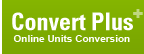# gram-force meter (Torque, Metric)

## 1 gram-force meter is equal to:Metric newton meter 0.00980665012480924 newton centimeter 0.980665012480924 dyne meter 980.665012480924 dyne centimeter 98066.5012480924 kilogram-force meter 0.001 kilogram-force centimeter 0.1 gram-force centimeter 100 British and U.S. long ton-force foot 3.229024e-06 short ton-force foot 3.6165069e-06 pound-force foot 0.0072330139 pound-force inch 0.086796166 ounce-force inch 1.3887387ConversionsAreaCapacity and VolumeCircular measureComputer storageCurrencyDistance and LengthEnergy and WorkFuel ConsumptionMass and WeightPowerPressureSpeedTemperatureTimeTorqueSearchLink to us